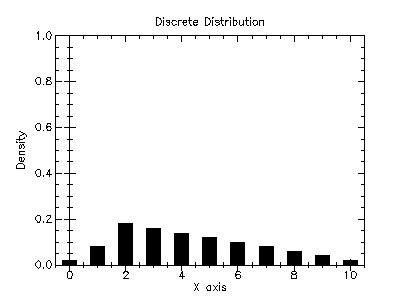Version: 4.2.1

### 7Random Number Distributions

This chapter describes the functions for generating random variates and computing their probability densities provided by the PLT Scheme Science Collection/

The functions described in this chapter are defined in the random-distributions sub-collection of the science collection. All of the modules in the random-distributions sub-collection can be made available using the form:

(require williams/science/random-distributions)

The random distribution graphics are provided as separate modules. To also include the random distribution graphics routines, use the following form:

(require williams/science/random-distributions-with-graphics)

The individual modules inthe random-distributions sub-collection can also be made available as described in the sections below.

#### 7.1The Beta DistributionBeta Distribution from Wolfram MathWorld.

The beta distribution functions are defined in the "beta.ss" file in the random-distributions subcollection of the science collection and are made available using the form:

 (require (planet williams/science/random-distributions/beta))

##### 7.1.1Random Variates from the Beta Distribution

 (random-beta s a b) → (real-in 0.0 1.0) s : random-source? a : real? b : real? (random-beta a b) → (real-in 0.0 1.0) a : real? b : real?
Returns a random variate from the beta distribution with parameters a and b using the random source s or (current-random-source) if s is not provided.

Example: Histogram of random variates from the beta distribution with parameters a = 2.0 and b = 3.0.

 #lang scheme (require (planet williams/science/random-distributions/beta)) (require (planet williams/science/histogram-with-graphics)) (let ((h (make-histogram-with-ranges-uniform 40 0.0 1.0))) (do ((i 0 (+ i 1))) ((= i 10000) (void)) (histogram-increment! h (random-beta 2.0 3.0))) (histogram-plot h "Histogram of the Beta Distribution"))

The following figure shows the resulting histogram: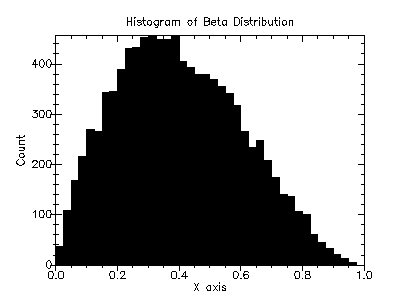##### 7.1.2Beta Distribution Density Functions

 (beta-pdf x a b) → (>=/c 0.0) x : real? a : real? b : real?
Computes the probability density, p(x), at x for the beta distribution with parameters a and b.

 (beta-cdf x a b) → (real-in 0.0 1.0) x : real? a : real? b : real?
Computes the cumulative density, d(x), at x for the beta distribution with parameters a and b.

##### 7.1.3Beta Distribution Graphics

The beta distribution graphics are defined in the "beta-graphics.ss" file in the random-distributions sub-collection of the science collection and are made available using the form:

 (require (planet williams/science/random-distributions/beta-graphics))

 (beta-plot a b) → any a : real? b : real?
Returns a plot of the probability density and cumulative density of the beta distribution with parameters a and b. The plot is produced by the plot collection provided with PLT Scheme.

Example: Plot of the probability density and cumulative density of the beta distribution with parameters a = 2.0 and b = 3.0.

 #lang scheme (require (planet williams/science/random-distributions/beta-graphics)) (beta-plot 2.0 3.0)

The following figure shows the resulting plot: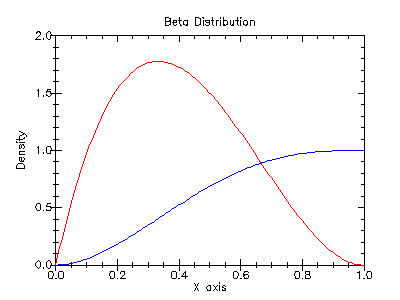#### 7.2The Bivariate Gaussian DistributionBivariate Normal Distribution from Wolfram MathWorld.

The bivariate Gaussian distribution functions are defined in the "bivariate-gaussian.ss" file in the random-distributions subcollection of the science collection and are made available using the form:

 (require (planet williams/science/random-distributions/bivariate-gaussian))

##### 7.2.1Random Variates from the Bivariate Haussian Distribution

(random-bivariate-gaussian s
sigma-x
sigma-y
rho)
 real? real?
s : random-source?
sigma-x : (>=/c 0.0)
sigma-y : (>=/c 0.0)
rho : (real-in -1.0 1.0)
(random-bivariate-gaussian sigma-x
sigma-y
rho)
 real? real?
sigma-x : (>=/c 0.0)
sigma-y : (>=/c 0.0)
rho : (real-in -1.0 1.0)
Returns a pair of correlated Gaussian variates, with mean 0, correlation coefficient rho, and standard deviations sigma-x and sigma-y in the x and y directions using the random source s or (current-random-source) if s is not provided. The correlation coefficient rho must lie between -1 and 1.

Example: 2D histogram of random variates from the bivariate Gaussian distribution with standard deviation 1.0 in both the x and y direction and correlation coefficient 0.0.

 #lang scheme (require (planet williams/science/random-distributions/bivariate)) (require (planet williams/science/histogram-2d-with-graphics)) (let ((h (make-histogram-2d-with-ranges-uniform 20 20 -3.0 3.0 -3.0 3.0))) (do ((i 0 (+ i 1))) ((= i 10000) (void)) (let-values (((x y) (random-bivariate-gaussian 1.0 1.0 0.0))) (histogram-2d-increment! h x y))) (histogram-2d-plot h "Histogram of the Bivariate Gaussian Distribution"))

The following figure shows the resulting histogram: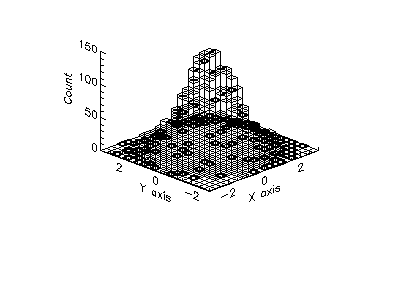##### 7.2.2Bivariate Gaussian Distribution Density Functions

 (bivariate-gaussian-pdf x y sigma-x sigma-y rho) → (>=/c 0.0)
x : real?
y : real?
sigma-x : (>=/c 0.0)
sigma-y : (>=/c 0.0)
rho : (real-in -1.0 1.0)
Computes the probability density, p(x, y), at (x, y) for the bivariate gaussian distribution with mean 0, correlation coefficient rho, and standard deviations sigma-x and sigma-y in the x and y directions.

##### 7.2.3Bivariate Gaussian Distribution Graphics

The bivariate Gaussian distribution graphics are defined in the "bivariate-gaussian-graphics.ss" file in the random-distributions sub-collection of the science collection and are made available using the form:

 (require (planet williams/science/random-distributions/bivariate-gaussian-graphics))

 (bivariate-plot sigma-x sigma-y rho) → any sigma-x : (>=/c 0.0) sigma-y : (>=/c 0.0) rho : (real-in -1.0 1.0)
Returns a plot of the probability density and cumulative density of the bivariate Gaussian distribution with mean 0, correlation coefficient rho, and standard deviations sigma-x and sigma-y in the x and y directions. The plot is produced by the plot collection provided with PLT Scheme.

Example: Plot of the probability density and cumulative density of the bivariate Gaussian distribution mean 0, correlation coefficient 0.0, and standard deviations 1.0 and 1.0 in the x and y directions.

 #lang scheme (require (planet williams/science/random-distributions/binomial-gaussian-graphics)) (bivariate-gaussian-plot 1.0 1.0 0.0)

The following figure shows the resulting plot: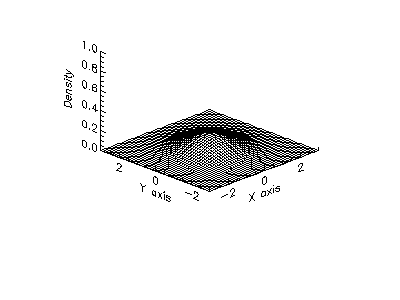#### 7.3The Chi-Squared DistributionChi-Squared Distribution from Wolfram MathWorld.

The chi-squared distribution functions are defined in the "chi-squared.ss" file in the random-distributions subcollection of the science collection and are made available using the form:

 (require (planet williams/science/random-distributions/chi-squared))

##### 7.3.1Random Variates from the Chi-Squared Distribution

 (random-chi-squared s nu) → (>=/c 0.0) s : random-source? nu : real? (random-chi-squared nu) → (>=/c 0.0) nu : real?
Returns a random variate from the chi-squared distribution with nu degrees of freedom using the random source s or (current-random-source) if s is not provided.

Example: Histogram of random variates from the chi-squared distribution with 3.0 degrees of freedom.

 #lang scheme (require (planet williams/science/random-distributions/chi-squared)) (require (planet williams/science/histogram-with-graphics)) (let ((h (make-histogram-with-ranges-uniform 40 0.0 10.0))) (do ((i 0 (+ i 1))) ((= i 10000) (void)) (histogram-increment! h (random-chi-squared 3.0))) (histogram-plot h "Histogram of the Chi-Squared Distribution"))

The following figure shows the resulting histogram: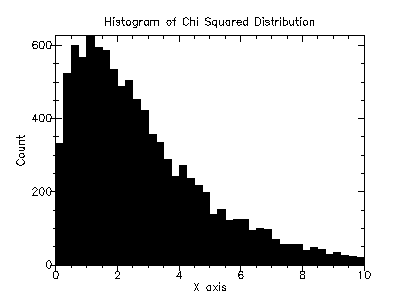##### 7.3.2Chi-Squared Distribution Density Functions

 (chi-squared-pdf x nu) → (>=/c 0.0) x : real? nu : real?
Computes the probability density, p(x), at x for the chi-squared distribution with nu degrees of freedom.

 (chi-squared-cdf x nu) → (real-in 0.0 1.0) x : real? nu : real?
Computes the cumulative density, d(x), at x for the chi-squared distribution with nu degrees of freedom.

##### 7.3.3Chi-Squared Distribution Graphics

The chi-squared distribution graphics are defined in the "chi-squared-graphics.ss" file in the random-distributions sub-collection of the science collection and are made available using the form:

 (require (planet williams/science/random-distributions/chi-squared-graphics))

 (chi-squared-plot nu) → any nu : real?
Returns a plot of the probability density and cumulative density of the chi-squared distribution with nu degrees of freedom. The plot is produced by the plot collection provided with PLT Scheme.

Example: Plot of the probability density and cumulative density of the chi-squared distribution with 3.0 degrees of freedom.

 #lang scheme (require (planet williams/science/random-distributions/chi-squared-graphics)) (chi-squared-plot 3.0)

The following figure shows the resulting plot: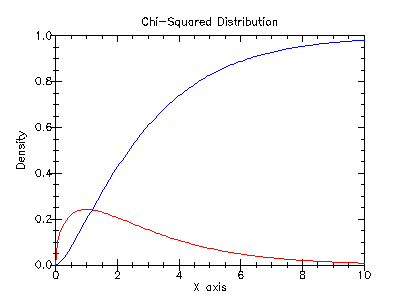#### 7.4The Exponential DistributionExponential Distribution from Wolfram MathWorld.

The exponential distribution functions are defined in the "exponential.ss" file in the random-distributions subcollection of the science collection and are made available using the form:

 (require (planet williams/science/random-distributions/exponential))

##### 7.4.1Random Variates from the Exponential Distribution

 (random-exponential s mu) → (>=/c 0.0) s : random-source? mu : (>/c 0.0) (random-chi-squared mu) → (>=/c 0.0) mu : (>/c 0.0)
Returns a random variate from the exponential distribution with mean mu using the random source s or (current-random-source) if s is not provided.

Example: Histogram of random variates from the exponential distribution with mean 1.0.

 #lang scheme (require (planet williams/science/random-distributions/exponential)) (require (planet williams/science/histogram-with-graphics)) (let ((h (make-histogram-with-ranges-uniform 40 0.0 8.0))) (do ((i 0 (+ i 1))) ((= i 10000) (void)) (histogram-increment! h (random-exponential 1.0))) (histogram-plot h "Histogram of the Exponential Distribution"))

The following figure shows the resulting histogram: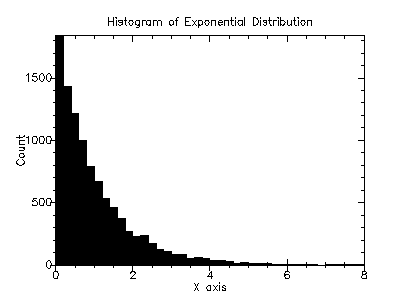##### 7.4.2Exponential Distribution Density Functions

 (exponential-pdf x mu) → (>=/c 0.0) x : real? mu : (>/c 0.0)
Computes the probability density, p(x), at x for the exponential distribution with mean mu.

 (exponential-cdf x mu) → (real-in 0.0 1.0) x : real? mu : (>/c 0.0)
Computes the cumulative density, d(x), at x for the exponential distribution with mean mu.

##### 7.4.3Exponential Distribution Graphics

The exponential distribution graphics are defined in the "exponential-graphics.ss" file in the random-distributions sub-collection of the science collection and are made available using the form:

 (require (planet williams/science/random-distributions/exponential-graphics))

 (exponential-plot mu) → any mu : (>/c 0.0)
Returns a plot of the probability density and cumulative density of the exponential distribution with mean mu. The plot is produced by the plot collection provided with PLT Scheme.

Example: Plot of the probability density and cumulative density of the exponential distribution with mean 3.0.

 #lang scheme (require (planet williams/science/random-distributions/exponential-graphics)) (exponential-plot 3.0)

The following figure shows the resulting plot: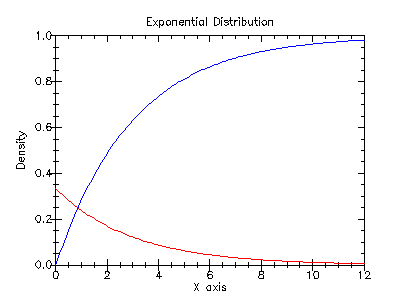#### 7.5The F-DistributionF-Distribution from Wolfram MathWorld.

The F-distribution functions are defined in the "f-distribution.ss" file in the random-distributions subcollection of the science collection and are made available using the form:

 (require (planet williams/science/random-distributions/f-distribution))

##### 7.5.1Random Variates from the F-Distribution

 (random-f-distribution s nu1 nu2) → (>=/c 0.0) s : random-source? nu1 : real? nu2 : real? (random-f-distribution nu1 nu2) → (>=/c 0.0) nu1 : real? nu2 : real?
Returns a random variate from the F-distribution with nu1 and nu2 degrees of freedom using the random source s or (current-random-source) if s is not provided.

Example: Histogram of random variates from the F-distribution with 2.0 and 3.0 degrees of freedom.

 #lang scheme (require (planet williams/science/random-distributions/f-distribution)) (require (planet williams/science/histogram-with-graphics)) (let ((h (make-histogram-with-ranges-uniform 40 0.0 10.0))) (do ((i 0 (+ i 1))) ((= i 10000) (void)) (histogram-increment! h (random-f-distribution 2.0 3.0))) (histogram-plot h "Histogram of the F-Distribution"))

The following figure shows the resulting histogram: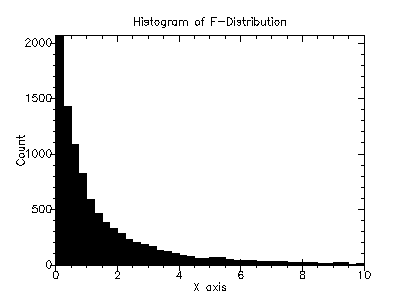##### 7.5.2F-Distribution Density Functions

 (f-distribution-pdf x nu1 nu2) → (>=/c 0.0) x : real? nu1 : real? nu2 : real?
Computes the probability density, p(x), at x for the F-distribution with nu1 and nu2 degrees of freedom.

 (f-distribution-cdf x nu1 nu2) → (real-in 0.0 1.0) x : real? nu1 : real? nu2 : real?
Computes the cumulative density, d(x), at x for the F-distribution with nu1 and nu2 degrees of freedom.

##### 7.5.3F-Distribution Graphics

The F-distribution graphics are defined in the "f-distribution-graphics.ss" file in the random-distributions sub-collection of the science collection and are made available using the form:

 (require (planet williams/science/random-distributions/f-distribution-graphics))

 (f-distribution-plot nu1 nu2) → any nu1 : real? nu2 : real?
Returns a plot of the probability density and cumulative density of the F-distribution with nu1 and nu2 degrees of freedom. The plot is produced by the plot collection provided with PLT Scheme.

Example: Plot of the probability density and cumulative density of the Fdistribution with 2.0 and 3.0 degrees of freedom.

 #lang scheme (require (planet williams/science/random-distributions/f-distribution-graphics)) (f-distribution-plot 2.0 3.0)

The following figure shows the resulting plot: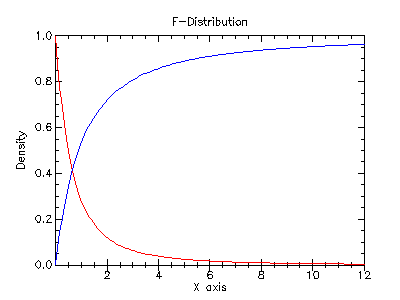#### 7.6The Flat (Uniform) DistributionUniform Distribution from Wolfram MathWorld.

The flat (uniform) distribution functions are defined in the "flat.ss" file in the random-distributions subcollection of the science collection and are made available using the form:

 (require (planet williams/science/random-distributions/flat))

Note that the name flat is used because uniform is already used for the more primitive random number functions in SRFI 27. Note that also matches the convention in the GNU Scientific Library [GSL].

##### 7.6.1Random Variates from the Flat (Uniform) Distribution

 (random-flat s a b) → real? s : random-source? a : real? b : (>/c a) (random-flat a b) → real? a : real? b : (>/c a)
Returns a random variate from the flat (uniform) distribution from a to b using the random source s or (current-random-source) if s is not provided.

Example: Histogram of random variates from the flat (uniform) distribution from 1.0 to 4.0.

 #lang scheme (require (planet williams/science/random-distributions/flat)) (require (planet williams/science/histogram-with-graphics)) (let ((h (make-histogram-with-ranges-uniform 40 1.0 4.0))) (do ((i 0 (+ i 1))) ((= i 10000) (void)) (histogram-increment! h (random-flat 1.0 4.0))) (histogram-plot h "Histogram of the Flat (Uniform) Distribution"))

The following figure shows the resulting histogram: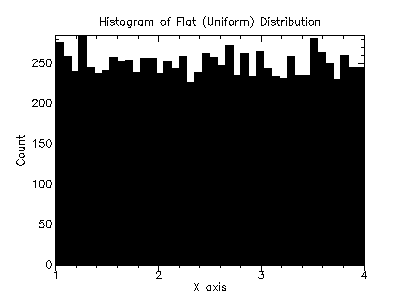##### 7.6.2Flat (Uniform) Distribution Density Functions

 (flat-pdf x a b) → (>=/c 0.0) x : real? a : real? b : (>/c a)
Computes the probability density, p(x), at x for the flat (uniform) distribution from a to b.

 (flat-cdf x a b) → (real-in 0.0 1.0) x : real? a : real? b : (>/c a)
Computes the cumulative density, d(x), at x for the flat (uniform) distribution from a to b.

##### 7.6.3Flat (Uniform) Distribution Graphics

The flat (uniform) distribution graphics are defined in the "flat-graphics.ss" file in the random-distributions sub-collection of the science collection and are made available using the form:

 (require (planet williams/science/random-distributions/flat-graphics))

 (flat-plot a b) → any a : real? b : (>/c a)
Returns a plot of the probability density and cumulative density of the flat (uniform) distribution from a to b. The plot is produced by the plot collection provided with PLT Scheme.

Example: Plot of the probability density and cumulative density of the flat (uniform) distribution from 1.0 to 4.0.

 #lang scheme (require (planet williams/science/random-distributions/flat-graphics)) (flat-plot 1.0 4.0)

The following figure shows the resulting plot: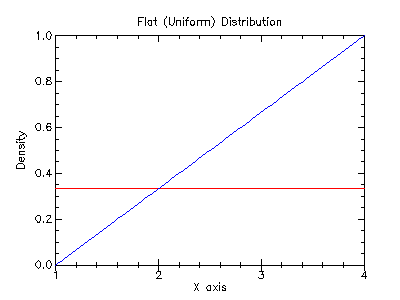#### 7.7The Gamma DistributionGamma Distribution from Wolfram MathWorld.

The gamma distribution functions are defined in the "gamma.ss" file in the random-distributions subcollection of the science collection and are made available using the form:

 (require (planet williams/science/random-distributions/gamma))

##### 7.7.1Random Variates from the Gamma Distribution

 (random-gamma s a b) → (>=/c 0.0) s : random-source? a : (>/c 0.0) b : real? (random-gamma a b) → (>=/c 0.0) a : (>/c 0.0) b : real?
Returns a random variate from the gamma distribution with parameters a and b using the random source s or (current-random-source) if s is not provided.

Example: Histogram of random variates from the gamma distribution with parameters 3.0 and 3.0.

 #lang scheme (require (planet williams/science/random-distributions/gamma)) (require (planet williams/science/histogram-with-graphics)) (let ((h (make-histogram-with-ranges-uniform 40 0.0 24.0))) (do ((i 0 (+ i 1))) ((= i 10000) (void)) (histogram-increment! h (random-gamma 3.0 3.0))) (histogram-plot h "Histogram of the Gamma Distribution"))

The following figure shows the resulting histogram: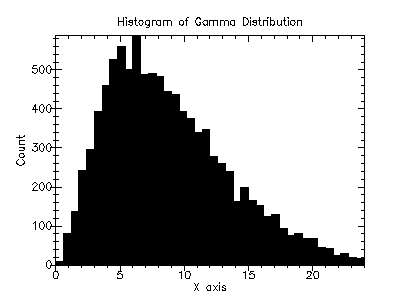##### 7.7.2Gamma Distribution Density Functions

 (gamma-pdf x a b) → (>=/c 0.0) x : real? a : (>=/c 0.0) b : real?
Computes the probability density, p(x), at x for the gamma distribution with parameters a and b.

 (gamma-cdf x a b) → (real-in 0.0 1.0) x : real? a : (>=/c 0.0) b : real?
Computes the cumulative density, d(x), at x for the gamma distribution with parameters a and b.

##### 7.7.3Gamma Distribution Graphics

The gamma distribution graphics are defined in the "gamma-graphics.ss" file in the random-distributions sub-collection of the science collection and are made available using the form:

 (require (planet williams/science/random-distributions/gamma-graphics))

 (gamma-plot a b) → any a : (>=/c 0.0) b : real?
Returns a plot of the probability density and cumulative density of the gamma distribution with parameters a and b. The plot is produced by the plot collection provided with PLT Scheme.

Example: Plot of the probability density and cumulative density of the gamma distribution with parameters 3.0 and 3.0.

 #lang scheme (require (planet williams/science/random-distributions/gamma-graphics)) (gamma-plot 3.0 3.0)

The following figure shows the resulting plot: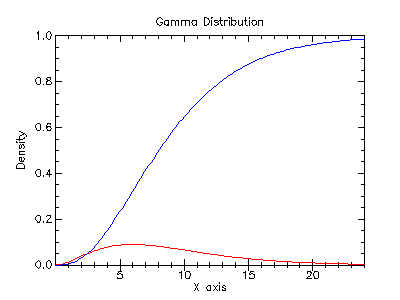#### 7.8The Gaussian (Normal) DistributionNormal Distribution from Wolfram MathWorld.

The Gaussian (normal) distribution functions are defined in the "gaussian.ss" file in the random-distributions subcollection of the science collection and are made available using the form:

 (require (planet williams/science/random-distributions/gaussian))

##### 7.8.1Random Variates from the Gaussian (Normal) Distribution

 (random-gaussian s mu sigma) → real? s : random-source? mu : real? sigma : (>=/c 0.0) (random-gaussian mu sigma) → real? mu : real? sigma : (>=/c 0.0)
Returns a random variate from the Gaussian (normal) distribution with mean mu and standard deviation sigma using the random source s or (current-random-source) if s is not provided. This function uses the Box-Mueller algorithm that requires two calls to the random source s.

Example: Histogram of random variates from the Gaussian (normal) distribution with mean 10.0 and standard deviation 2.0.

 #lang scheme (require (planet williams/science/random-distributions/gaussian)) (require (planet williams/science/histogram-with-graphics)) (let ((h (make-histogram-with-ranges-uniform 40 4.0 16.0))) (do ((i 0 (+ i 1))) ((= i 10000) (void)) (histogram-increment! h (random-gaussian 10.0 2.0))) (histogram-plot h "Histogram of the Gaussian (Normal) Distribution"))

The following figure shows the resulting histogram: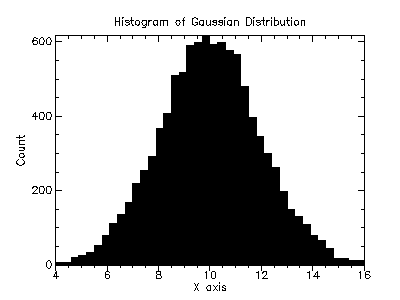(random-unit-gaussian s) → real? s : random-source? (random-unit-gaussian) → real?
Returns a random variate from the Gaussian (normal) distribution with mean 0.0 and standard deviation 1.0 using the random source s or (current-random-source) if s is not provided. This function uses the Box-Mueller algorithm that requires two calls to the random source s.

Example: Histogram of random variates from the unit Gaussian (normal) distribution.

 #lang scheme (require (planet williams/science/random-distributions/gaussian)) (require (planet williams/science/histogram-with-graphics)) (let ((h (make-histogram-with-ranges-uniform 40 -3.0 3.0))) (do ((i 0 (+ i 1))) ((= i 10000) (void)) (histogram-increment! h (random-unit-gaussian))) (histogram-plot h "Histogram of the Unit Gaussian (Normal) Distribution"))

The following figure shows the resulting histogram: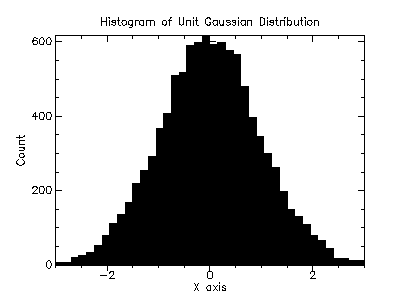(random-gaussian-ratio-method s mu sigma) → real? s : random-source? mu : real? sigma : (>=/c 0.0) (random-gaussian-ratio-method mu sigma) → real? mu : real? sigma : (>=/c 0.0)
Returns a random variate from the Gaussian (normal) distribution with mean mu and standard deviation sigma using the random source s or (current-random-source) if s is not provided. This function uses the Kinderman-Monahan ratio method.

 (random-unit-gaussian-ratio-method s) → real? s : random-source? (random-unit-gaussian-ratio-method) → real?
Returns a random variate from the Gaussian (normal) distribution with mean 0.0 and standard deviation 1.0 using the random source s or (current-random-source) if s is not provided. This function uses the Kinderman-Monahan ratio method.

##### 7.8.2Gaussian (Normal) Distribution Density Functions

 (gaussian-pdf x mu sigma) → (>=/c 0.0) x : real? mu : real? sigma : (>=/c 0.0)
Computes the probability density, p(x), at x for the Gaussian (normal) distribution with mean mu and standard deviation sigma.

 (gaussian-cdf x mu sigma) → (real-in 0.0 1.0) x : real? mu : real? sigma : (>=/c 0.0)
Computes the cumulative density, d(x), at x for the Gaussian (normal) distribution with mean mu and standard deviation sigma.

 (unit-gaussian-pdf x) → (>=/c 0.0) x : real?
Computes the probability density, p(x), at x for the unit Gaussian (normal) distribution.

 (unit-gaussian-cdf x) → (real-in 0.0 1.0) x : real?
Computes the cumulative density, d(x), at x for the unit Gaussian (normal) distribution.

##### 7.8.3Gaussian (Normal) Distribution Graphics

The Gaussian (normal) distribution graphics are defined in the "gaussian-graphics.ss" file in the random-distributions sub-collection of the science collection and are made available using the form:

 (require (planet williams/science/random-distributions/gaussian-graphics))

 (gassian-plot mu sigma) → any mu : real? sigma : (>=/c 0.0)
Returns a plot of the probability density and cumulative density of the Gaussian (normal) distribution with mean mu and standard deviation sigma. The plot is produced by the plot collection provided with PLT Scheme.

Example: Plot of the probability density and cumulative density of the Gaussian (normal) distribution with parameters mean 10.0 and standard deviation 2.0.

 #lang scheme (require (planet williams/science/random-distributions/gaussian-graphics)) (gaussian-plot 10.0 2.0)

The following figure shows the resulting plot: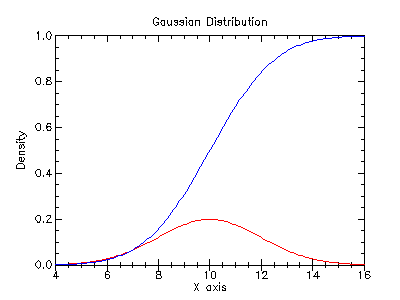(unit-gassian-plot) → any
Returns a plot of the probability density and cumulative density of the unit Gaussian (normal) distribution. The plot is produced by the plot collection provided with PLT Scheme.

Example: Plot of the probability density and cumulative density of the unit Gaussian (normal) distribution.

 #lang scheme (require (planet williams/science/random-distributions/gaussian-graphics)) (unit-gaussian-plot)

The following figure shows the resulting plot: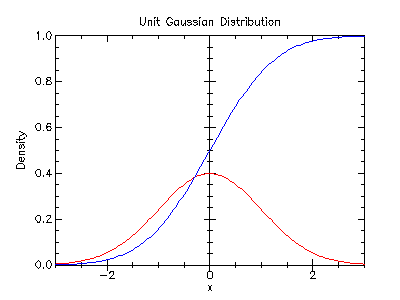#### 7.9The Gaussian Tail Distribution

The Gaussian tail distribution functions are defined in the "gaussian-tail.ss" file in the random-distributions subcollection of the science collection and are made available using the form:

 (require (planet williams/science/random-distributions/gaussian-tail))

##### 7.9.1Random Variates from the Gaussian Tail Distribution

 (random-gaussian-tail s a mu sigma) → real? s : random-source? a : real? mu : real? sigma : (>=/c 0.0) (random-gaussian-tail a mu sigma) → real? a : real? mu : real? sigma : (>=/c 0.0)
Returns a random variate from the upper tail of the Gaussian distribution with mean mu and standard deviation sigma using the random source s or (current-random-source) if s is not provided. The value returned is larger than the lower limit a, which must be greater than the mean mu.

Example: Histogram of random variates from the upper tail greater than 16.0 of the Gaussian distribution with mean 10.0 and standard deviation 2.0.

 #lang scheme (require (planet williams/science/random-distributions/gaussian)) (require (planet williams/science/histogram-with-graphics)) (let ((h (make-histogram-with-ranges-uniform 40 16.0 22.0))) (do ((i 0 (+ i 1))) ((= i 10000) (void)) (histogram-increment! h (random-gaussian-tail 16.0 10.0 2.0))) (histogram-plot h "Histogram of the Gaussian Tail Distribution"))

The following figure shows the resulting histogram: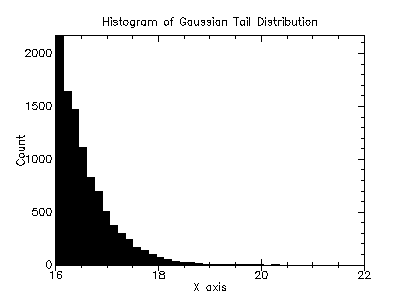(random-unit-gaussian-tail s a) → real? s : random-source? a : (>/c 0.0) (random-unit-gaussian-tail a) → real? a : (>/c 0.0)
Returns a random variate from the upper tail of the Gaussian distribution with mean 0 and standard deviation 1 using the random source s or (current-random-source) if s is not provided. The value returned is larger than the lower limit a, which must be greater than the mean mu.

##### 7.9.2Gaussian Tail Distribution Density Functions

 (gaussian-tail-pdf x a mu sigma) → (>=/c 0.0) x : real? a : real? mu : real? sigma : (>=/c 0.0)
Computes the probability density, p(x), at x for the upper tail greater than a of the Gaussian distribution with mean mu and standard deviation sigma.

 (unit-gaussian-tail-pdf x a) → (>=/c 0.0) x : real? a : (>/c 0.0)
Computes the probability density, p(x), at x for the upper tail greater than a of the unit Gaussian distribution.

##### 7.9.3Gaussian Tail Distribution Graphics

The Gaussian tail distribution graphics are defined in the "gaussian-tail-graphics.ss" file in the random-distributions sub-collection of the science collection and are made available using the form:

 (require (planet williams/science/random-distributions/gaussian-tail-graphics))

 (gassian-tail-plot a mu sigma) → any a : real? mu : real? sigma : (>=/c 0.0)
Returns a plot of the probability density and cumulative density of the upper tail greater than a of the Gaussian distribution with mean mu and standard deviation sigma. The plot is produced by the plot collection provided with PLT Scheme.

Example: Plot of the probability density and cumulative density of the upper tail greater than 16.0 of the Gaussian distribution with parameters mean 10.0 and standard deviation 2.0.

 #lang scheme (require (planet williams/science/random-distributions/gaussian-tail-graphics)) (gaussian-plot 16.0 10.0 2.0)

The following figure shows the resulting plot: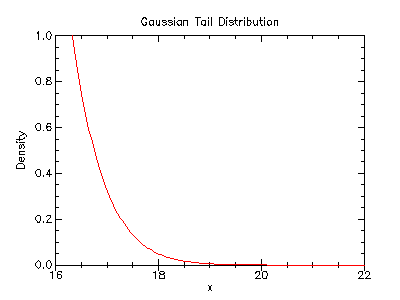(unit-gassian-tail-plot a) → any a : (>/c 0.0)
Returns a plot of the probability density and cumulative density of the upper tail greater than a of the unit Gaussian distribution. The plot is produced by the plot collection provided with PLT Scheme.

Example: Plot of the probability density and cumulative density of the upper tail greater than 3.0 of the unit Gaussian (normal) distribution.

 #lang scheme (require (planet williams/science/random-distributions/gaussian-tail-graphics)) (unit-gaussian-tail-plot 3.0)

The following figure shows the resulting plot: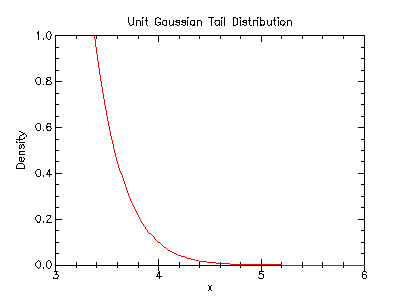#### 7.10The Log Normal DistributionLog Normal Distribution from Wolfram MathWorld.

The log normal distribution functions are defined in the "lognormal.ss" file in the random-distributions subcollection of the science collection and are made available using the form:

 (require (planet williams/science/random-distributions/lognormal))

##### 7.10.1Random Variates from the Log Normal Distribution

 (random-lognormal s mu sigma) → real? s : random-source? mu : real? sigma : (>=/c 0.0) (random-lognormal mu sigma) → real? mu : real? sigma : (>=/c 0.0)
Returns a random variate from the log normal distribution with mean mu and standard deviation sigma using the random source s or (current-random-source) if s is not provided.

Example: Histogram of random variates from the log normal distribution with parameters mean 0.0 and standard deviation 1.0.

 #lang scheme (require (planet williams/science/random-distributions/lognormal)) (require (planet williams/science/histogram-with-graphics)) (let ((h (make-histogram-with-ranges-uniform 40 0.0 6.0))) (do ((i 0 (+ i 1))) ((= i 10000) (void)) (histogram-increment! h (random-log-normal 0.0 1.0))) (histogram-plot h "Histogram of the Log Normal Distribution"))

The following figure shows the resulting histogram: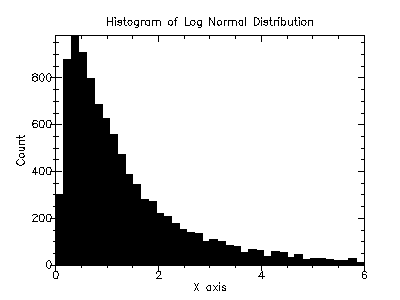##### 7.10.2Log Normal Distribution Density Functions

 (lognormal-pdf x mu sigma) → (>=/c 0.0) x : real? mu : real? sigma : (>=/c 0.0)
Computes the probability density, p(x), at x for the log normal distribution with mean mu and standard deviation sigma.

 (lognormal-cdf x mu sigma) → (real-in 0.0 1.0) x : real? mu : real? sigma : (>=/c 0.0)
Computes the cumulative density, d(x), at x for the log normal distribution with mean mu and standard deviation sigma.

##### 7.10.3Log Normal Distribution Graphics

The log normal distribution graphics are defined in the "lognormal-graphics.ss" file in the random-distributions sub-collection of the science collection and are made available using the form:

 (require (planet williams/science/random-distributions/lognormal-graphics))

 (lognormal-plot mu sigma) → any mu : real? sigma : (>=/c 0.0)
Returns a plot of the probability density and cumulative density of the log normal distribution with mean mu and standard deviation sigma. The plot is produced by the plot collection provided with PLT Scheme.

Example: Plot of the probability density and cumulative density of the log normal distribution with mean 0.0 and standard deviation 1.0.

 #lang scheme (require (planet williams/science/random-distributions/lognormal-graphics)) (lognormal-plot 0.0 1.0)

The following figure shows the resulting plot: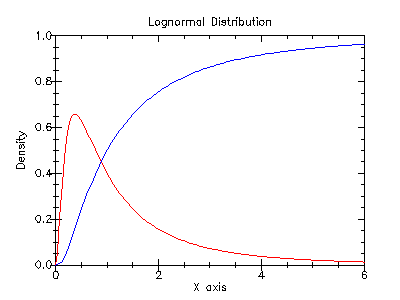#### 7.11The Pareto DistributionPareto Distribution from Wolfram MathWorld.

The Pareto distribution functions are defined in the "pareto.ss" file in the random-distributions subcollection of the science collection and are made available using the form:

 (require (planet williams/science/random-distributions/pareto))

##### 7.11.1Random Variates from the Pareto Distribution

 (random-pareto s a b) → real? s : random-source? a : real? b : real? (random-pareto a b) → real? a : real? b : real?
Returns a random variate from the Pareto distribution with parameters a and b using the random source s or (current-random-source) if s is not provided.

Example: Histogram of random variates from the Pareto distribution with parameters a = 2.0 and b = 3.0.

 #lang scheme (require (planet williams/science/random-distributions/pareto)) (require (planet williams/science/histogram-with-graphics)) (let ((h (make-histogram-with-ranges-uniform 40 1.0 21.0))) (do ((i 0 (+ i 1))) ((= i 10000) (void)) (histogram-increment! h (random-pareto 1.0 1.0))) (histogram-plot h "Histogram of the Pareto Distribution"))

The following figure shows the resulting histogram: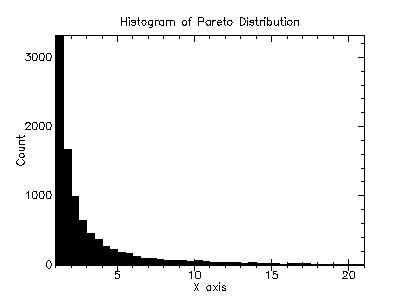##### 7.11.2Pareto Distribution Density Functions

 (pareto-pdf x a b) → (>=/c 0.0) x : real? a : real? b : real?
Computes the probability density, p(x), at x for the Pareto distribution with parameters a and b.

 (pareto-cdf x a b) → (real-in 0.0 1.0) x : real? a : real? b : real?
Computes the cumulative density, d(x), at x for the Pareto distribution with parameters a and b.

##### 7.11.3Pareto Distribution Graphics

The Pareto distribution graphics are defined in the "pareto-graphics.ss" file in the random-distributions sub-collection of the science collection and are made available using the form:

 (require (planet williams/science/random-distributions/pareto-graphics))

 (pareto-plot a b) → any a : real? b : real?
Returns a plot of the probability density and cumulative density of the Pareto distribution with parameters a and b. The plot is produced by the plot collection provided with PLT Scheme.

Example: Plot of the probability density and cumulative density of the Pareto distribution with parameters a = 1.0 and b = 1.0.

 #lang scheme (require (planet williams/science/random-distributions/pareto-graphics)) (beta-plot 1.0 1.0)

The following figure shows the resulting plot: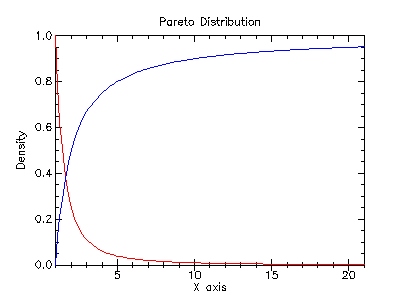#### 7.12The t-DistributionStudent’s t-Distribution from Wolfram MathWorld.

The t-distribution functions are defined in the "t-distribution.ss" file in the random-distributions subcollection of the science collection and are made available using the form:

 (require (planet williams/science/random-distributions/t-distribution))

##### 7.12.1Random Variates from the t-Distribution

 (random-t-distribution s nu) → real? s : random-source? nu : real? (random-t-distribution nu) → real? nu : real?
Returns a random variate from the t-distribution with nu degrees of freedom using the random source s or (current-random-source) if s is not provided.

Example: Histogram of random variates from the t-distribution with 1.0 degrees of freedom.

 #lang scheme (require (planet williams/science/random-distributions/t-distribution)) (require (planet williams/science/histogram-with-graphics)) (let ((h (make-histogram-with-ranges-uniform 40 -6.0 6.0))) (do ((i 0 (+ i 1))) ((= i 10000) (void)) (histogram-increment! h (random-t-distribution 1.0))) (histogram-plot h "Histogram of the t-Distribution"))

The following figure shows the resulting histogram: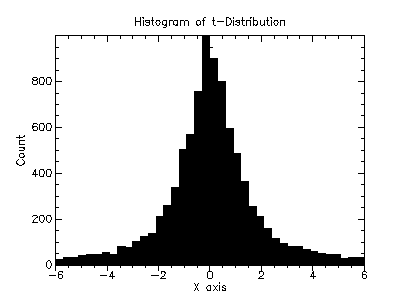##### 7.12.2t-Distribution Density Functions

 (t-distribution-pdf x nu) → (>=/c 0.0) x : real? nu : real?
Computes the probability density, p(x), at x for the t-distribution with nu degrees of freedom.

 (t-distribution-cdf x nu) → (real-in 0.0 1.0) x : real? nu : real?
Computes the cumulative density, d(x), at x for the t-distribution with nu degrees of freedom.

##### 7.12.3t-Distribution Graphics

The t-distribution graphics are defined in the "t-distribution-graphics.ss" file in the random-distributions sub-collection of the science collection and are made available using the form:

 (require (planet williams/science/random-distributions/t-distribution-graphics))

 (t-distribution-plot nu) → any nu : real?
Returns a plot of the probability density and cumulative density of the t-distribution with nu degrees of freedom. The plot is produced by the plot collection provided with PLT Scheme.

Example: Plot of the probability density and cumulative density of the t-distribution with 1.0 degrees of freedom.

 #lang scheme (require (planet williams/science/random-distributions/t-distribution-graphics)) (t-distribution-plot 1.0)

The following figure shows the resulting plot: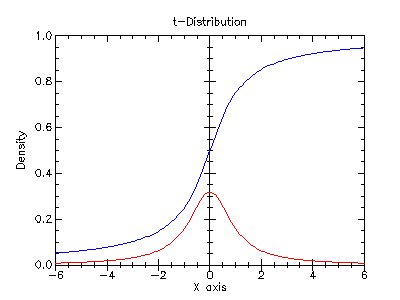#### 7.13The Triangular DistributionTriangular Distribution from Wolfram MathWorld.

The triangular distribution functions are defined in the "triangular.ss" file in the random-distributions subcollection of the science collection and are made available using the form:

 (require (planet williams/science/random-distributions/triangular))

##### 7.13.1Random Variates from the Triangular Distribution

 (random-triangular s a b c) → real? s : random-source? a : real? b : (>/c a) c : (real-in a b) (random-triangular a b c) → real? a : real? b : (>/c a) c : (real-in a b)
Returns a random variate from the triangular distribution with minimum value a, maximum value b, and most likely value c using the random source s or (current-random-source) if s is not provided.

Example: Histogram of random variates from the triangular distribution with minimum value 1.0, maximum value 4.0, and most likely value 2.0.

 #lang scheme (require (planet williams/science/random-distributions/triangular)) (require (planet williams/science/histogram-with-graphics)) (let ((h (make-histogram-with-ranges-uniform 40 1.0 4.0))) (do ((i 0 (+ i 1))) ((= i 10000) (void)) (histogram-increment! h (random-traingular 1.0 4.0 2.0))) (histogram-plot h "Histogram of the Triangular Distribution"))

The following figure shows the resulting histogram: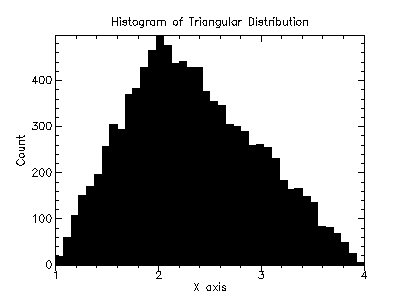##### 7.13.2Triangular Distribution Density Functions

 (triangular-pdf x a b c) → (>=/c 0.0) x : real? a : real? b : (>/c a) c : (real-in a b)
Computes the probability density, p(x), at x for the triangular distribution with minimum value a, maximum value b, and most likely value c.

 (triangular-cdf x a b c) → (real-in 0.0 1.0) x : real? a : real? b : (>/c a) c : (real-in a b)
Computes the cumulative density, d(x), at x for the triangular distribution with minimum value a, maximum value b, and most likely value c.

##### 7.13.3Triangular Distribution Graphics

The triangular distribution graphics are defined in the "triangular-graphics.ss" file in the random-distributions sub-collection of the science collection and are made available using the form:

 (require (planet williams/science/random-distributions/triangular-graphics))

 (triangular-plot a b c) → any a : real? b : (>/c a) c : (real-in a b)
Returns a plot of the probability density and cumulative density of the triangular distribution with minimum value a, maximum value b, and most likely value c. The plot is produced by the plot collection provided with PLT Scheme.

Example: Plot of the probability density and cumulative density of the triangular distribution with minimum value 1.0, maximum value 4.0, and most likely value 2.0.

 #lang scheme (require (planet williams/science/random-distributions/triangular-graphics)) (triangular-plot 1.0)

The following figure shows the resulting plot: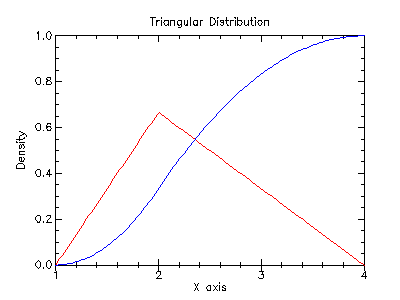#### 7.14The Bernoulli DistributionBernoulli Distribution from Wolfram MathWorld.

The Bernoulli distribution functions are defined in the "bernoulli.ss" file in the random-distributions subcollection of the science collection and are made available using the form:

 (require (planet williams/science/random-distributions/bernoulli))

##### 7.14.1Random Variates from the Bernoulli Distribution

 (random-bernoulli s p) → (integer-in 0 1) s : random-source? p : (real-in 0.0 1.0) (random-bernoulli p) → (integer-in 0 1) p : (real-in 0.0 1.0)
Returns a random variate from the Bernoulli distribution with probability p using the random source s or (current-random-source) if s is not provided.

Example: Histogram of random variates from the Bernoulli distribution with probability 0.6.

 #lang scheme (require (planet williams/science/random-distributions/bernoulli)) (require (planet williams/science/discrete-histogram-with-graphics)) (let ((h (make-discrete-histogram))) (do ((i 0 (+ i 1))) ((= i 10000) (void)) (discrete-histogram-increment! h (random-bernoulli 0.6))) (histogram-plot h "Histogram of the Bernoulli Distribution"))

The following figure shows the resulting histogram: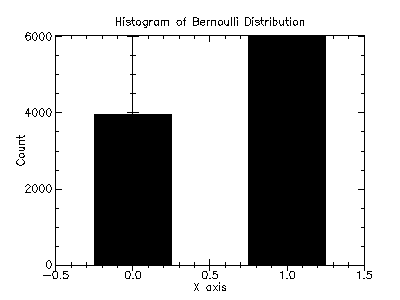##### 7.14.2Bernoulli Distribution Density Functions

 (bernoulli-pdf k p) → (>=/c 0.0) k : integer? p : (real-in 0.0 1.0)
Computes the probability density, p(k), at k for the Bernoulli distribution with probability p.

 (bernoulli-cdf k p) → (real-in 0.0 1.0) k : integer? p : (real-in 0.0 1.0)
Computes the cumulative density, d(k), at k for the Bernoulli distribution with probability p.

##### 7.14.3Bernoulli Distribution Graphics

The Bernoulli distribution graphics are defined in the "bernoulli-graphics.ss" file in the random-distributions sub-collection of the science collection and are made available using the form:

 (require (planet williams/science/random-distributions/bernoulli-graphics))

 (bernoulli-plot p) → any p : (real-in 0.0 1.0)
Returns a plot of the probability density and cumulative density of the Bernoulli distribution with probability p. The plot is produced by the plot collection provided with PLT Scheme.

Example: Plot of the probability density and cumulative density of the Bernoulli distribution with probability 0.6.

 #lang scheme (require (planet williams/science/random-distributions/bernoulli-graphics)) (bernoulli-plot 0.6)

The following figure shows the resulting plot: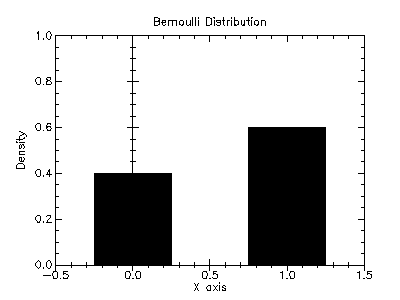#### 7.15The Binomial DistributionBinomial Distribution from Wolfram MathWorld.

The binomial distribution functions are defined in the "binomial.ss" file in the random-distributions subcollection of the science collection and are made available using the form:

 (require (planet williams/science/random-distributions/binomial))

##### 7.15.1Random Variates from the Binomial Distribution

 (random-binomial s p n) → natural-number/c s : random-source? p : (real-in 0.0 1.0) n : natural-number/c (random-binomial p n) → natural-number/c p : (real-in 0.0 1.0) n : natural-number/c
Returns a random variate from the binomial distribution with parameters p and n using the random source s or (current-random-source) if s is not provided.

Example: Histogram of random variates from the binomial distribution with parameters 0.5 and 20.

 #lang scheme (require (planet williams/science/random-distributions/binomial)) (require (planet williams/science/discrete-histogram-with-graphics)) (let ((h (make-discrete-histogram))) (do ((i 0 (+ i 1))) ((= i 10000) (void)) (discrete-histogram-increment! h (random-bernoulli 0.5 20))) (histogram-plot h "Histogram of the Binomial Distribution"))

The following figure shows the resulting histogram: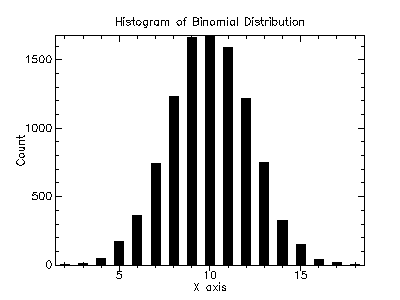##### 7.15.2Binomial Distribution Density Functions

 (bnomial-pdf k p n) → (>=/c 0.0) k : integer? p : (real-in 0.0 1.0) n : natural-number/c
Computes the probability density, p(k), at k for the binomial distribution with parameters p and n.

##### 7.15.3Binomial Distribution Graphics

The binomial distribution graphics are defined in the "binomial-graphics.ss" file in the random-distributions sub-collection of the science collection and are made available using the form:

 (require (planet williams/science/random-distributions/binomial-graphics))

 (binomial-plot p n) → any p : (real-in 0.0 1.0) n : natural-number/c
Returns a plot of the probability density and cumulative density of the binomial distribution with parameters p and n. The plot is produced by the plot collection provided with PLT Scheme.

Example: Plot of the probability density and cumulative density of the binomial distribution with parameters 0.5 and 20.

 #lang scheme (require (planet williams/science/random-distributions/binomial-graphics)) (bernoulli-plot 0.5 20)

The following figure shows the resulting plot: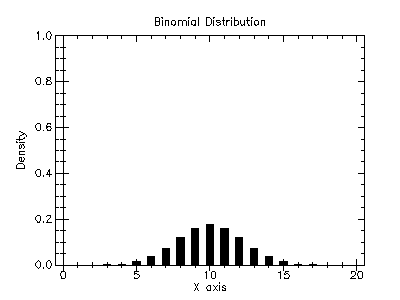#### 7.16The Geometric DistributionGeometric Distribution from Wolfram MathWorld.

The geometric distribution functions are defined in the "geometric.ss" file in the random-distributions subcollection of the science collection and are made available using the form:

 (require (planet williams/science/random-distributions/geometric))

##### 7.16.1Random Variates from the Geometric Distribution

 (random-geometric s p) → natural-number/c s : random-source? p : (real-in 0.0 1.0) (random-geometric p) → natural-numer/c p : (real-in 0.0 1.0)
Returns a random variate from the geometric distribution with probability p using the random source s or (current-random-source) if s is not provided.

Example: Histogram of random variates from the geometric distribution with probability 0.5.

 #lang scheme (require (planet williams/science/random-distributions/geometric)) (require (planet williams/science/discrete-histogram-with-graphics)) (let ((h (make-discrete-histogram))) (do ((i 0 (+ i 1))) ((= i 10000) (void)) (discrete-histogram-increment! h (random-geometric 0.5))) (histogram-plot h "Histogram of the Geometric Distribution"))

The following figure shows the resulting histogram: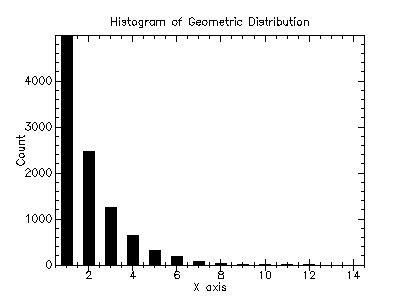##### 7.16.2Geometric Distribution Density Functions

 (geometric-pdf k p) → (>=/c 0.0) k : integer? p : (real-in 0.0 1.0)
Computes the probability density, p(k), at k for the geometric distribution with probability p.

##### 7.16.3Geometric Distribution Graphics

The geometric distribution graphics are defined in the "geometric-graphics.ss" file in the random-distributions sub-collection of the science collection and are made available using the form:

 (require (planet williams/science/random-distributions/geometric-graphics))

 (geometric-plot p) → any p : (real-in 0.0 1.0)
Returns a plot of the probability density and cumulative density of the geometric distribution with probability p. The plot is produced by the plot collection provided with PLT Scheme.

Example: Plot of the probability density and cumulative density of the geometric distribution with probability 0.5.

 #lang scheme (require (planet williams/science/random-distributions/geometric-graphics)) (geometric-plot 0.5)

The following figure shows the resulting plot: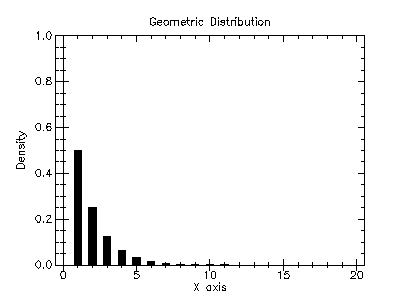#### 7.17The Logarithmic Distribution

Note that the logarithmic distribution in the GSL, and as implemented in the science collection, is a discrete version of the exponential distribution.

The logarithmic distribution functions are defined in the "logarithmic.ss" file in the random-distributions subcollection of the science collection and are made available using the form:

 (require (planet williams/science/random-distributions/logarithmetic))

##### 7.17.1Random Variates from the Logarithmic Distribution

 (random-logarithmic s p) → natural-number/c s : random-source? p : (real-in 0.0 1.0) (random-logarithmic p) → natural-number/c p : (real-in 0.0 1.0)
Returns a random variate from the logarithmic distribution with probability p using the random source s or (current-random-source) if s is not provided.

Example: Histogram of random variates from the logarithmic distribution with probability 0.5.

 #lang scheme (require (planet williams/science/random-distributions/logarithmic)) (require (planet williams/science/discrete-histogram-with-graphics)) (let ((h (make-discrete-histogram))) (do ((i 0 (+ i 1))) ((= i 10000) (void)) (discrete-histogram-increment! h (random-logarithmic 0.5))) (histogram-plot h "Histogram of the Logarithmic Distribution"))

The following figure shows the resulting histogram: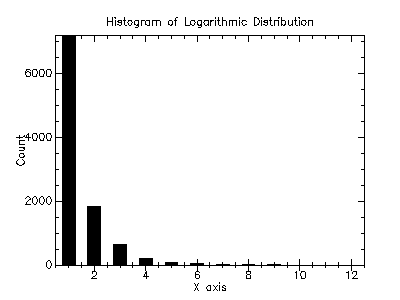##### 7.17.2Logarithmic Distribution Density Functions

 (logarithmic-pdf k p) → (>=/c 0.0) k : integer? p : (real-in 0.0 1.0)
Computes the probability density, p(k), at k for the logarithmic distribution with probability p.

##### 7.17.3Logarithmic Distribution Graphics

The logarithmic distribution graphics are defined in the "logarithmic-graphics.ss" file in the random-distributions sub-collection of the science collection and are made available using the form:

 (require (planet williams/science/random-distributions/logarithmic-graphics))

 (logarithmic-plot p) → any p : (real-in 0.0 1.0)
Returns a plot of the probability density and cumulative density of the logarithmic distribution with probability p. The plot is produced by the plot collection provided with PLT Scheme.

Example: Plot of the probability density and cumulative density of the logarithmic distribution with probability 0.5.

 #lang scheme (require (planet williams/science/random-distributions/logarithmic-graphics)) (logarithmic-plot 0.5)

The following figure shows the resulting plot: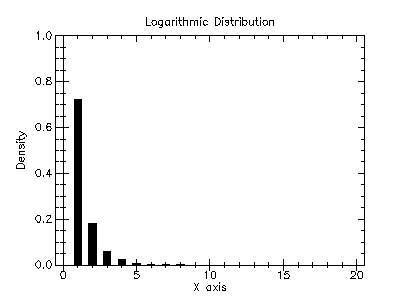#### 7.18The Poisson DistributionPoisson Distribution from Wolfram MathWorld.

The Poisson distribution functions are defined in the "poisson.ss" file in the random-distributions subcollection of the science collection and are made available using the form:

 (require (planet williams/science/random-distributions/poisson))

##### 7.18.1Random Variates from the Poisson Distribution

 (random-poisson s mu) → natural-number/c s : random-source? mu : real? (random-poisson mu) → natural-number/c mu : real?
Returns a random variate from the Poisson distribution with mean mu using the random source s or (current-random-source) if s is not provided.

Example: Histogram of random variates from the Poisson distribution with mean 10.0.

 #lang scheme (require (planet williams/science/random-distributions/poisson)) (require (planet williams/science/discrete-histogram-with-graphics)) (let ((h (make-discrete-histogram))) (do ((i 0 (+ i 1))) ((= i 10000) (void)) (discrete-histogram-increment! h (random-poisson 10.0))) (histogram-plot h "Histogram of the Poisson Distribution"))

The following figure shows the resulting histogram: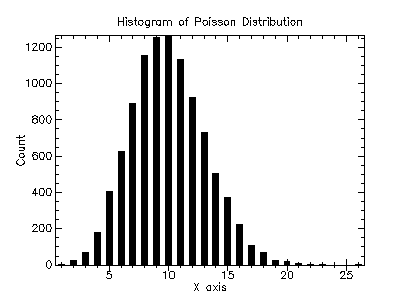##### 7.18.2Poisson Distribution Density Functions

 (poisson-pdf k mu) → (>=/c 0.0) k : integer? mu : real?
Computes the probability density, p(k), at k for the Poisson distribution with mean mu.

##### 7.18.3Poisson Distribution Graphics

The Poisson distribution graphics are defined in the "poisson-graphics.ss" file in the random-distributions sub-collection of the science collection and are made available using the form:

 (require (planet williams/science/random-distributions/poisson-graphics))

 (poisson-plot mu) → any mu : real?
Returns a plot of the probability density and cumulative density of the Poisson distribution with mean mu. The plot is produced by the plot collection provided with PLT Scheme.

Example: Plot of the probability density and cumulative density of the Poisson distribution with mean 10.0.

 #lang scheme (require (planet williams/science/random-distributions/poisson-graphics)) (poisson-plot 10.0)

The following figure shows the resulting plot: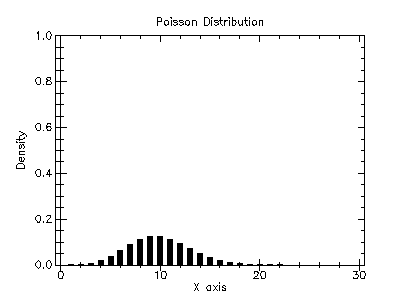#### 7.19General Discrete Distributions

The discrete distribution functions are defined in the "discrete.ss" file in the random-distributions subcollection of the science collection and are made available using the form:

 (require (planet williams/science/random-distributions/discrete))

##### 7.19.1Creating Discrete Distributions

 (make-discrete weights) → discrete? weights : (vector-of real?)
Returns a discrete distribution whose probability density is given by the specified weights. Note that the weights do not have to sum to one.

##### 7.19.2Random Variates from a Discrete Distribution

 (random-discrete s d) → integer? s : random-source? d : discrete? (random-discrete d) → integer? d : discrete?
Returns a random variate from a general discrete distribution d using the random source s or (current-random-source) if s is not provided.

Example: Histogram of random variates from a discrete distribution with weights 0.1, 0.4, 0.9, 0.8, 0.7, 0.6, 0.5, 0.4, 0.3, 0.2, and 0.1.

 #lang scheme (require (planet williams/science/random-distributions/discrete)) (require (planet williams/science/discrete-histogram-with-graphics)) (let ((h (make-discrete-histogram)) (d (make-discrete #(0.1 0.4 0.9 0.8 0.7 0.6 0.5 0.4 0.3 0.2 0.1)))) (do ((i 0 (+ i 1))) ((= i 10000) (void)) (discrete-histogram-increment! h (random-discrete d))) (histogram-plot h "Histogram of a Discrete Distribution"))

The following figure shows the resulting histogram: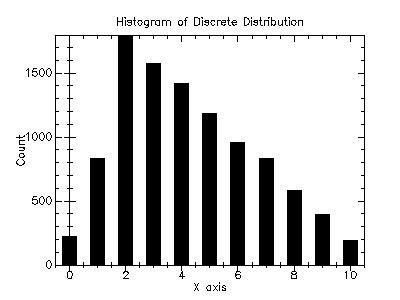##### 7.19.3Discrete Distribution Density Functions

 (discrete-pdf d k) → (real-in 0.0 1.0) d : discrete? k : integer?
Computes the probability density, p(k), at k for a discrete distribution d.

 (discrete-cdf d k) → (real-in 0.0 1.0) d : discrete? k : integer?
Computes the cumulative density, d(k), at k for a discrete distribution d.

##### 7.19.4Discrete Distribution Graphics

The discrete distribution graphics are defined in the "discrete-graphics.ss" file in the random-distributions sub-collection of the science collection and are made available using the form:

 (require (planet williams/science/random-distributions/discrete-graphics))

 (discrete-plot d) → any d : discrete?
Returns a plot of the probability density and cumulative density of a discrete distribution d. The plot is produced by the plot collection provided with PLT Scheme.

Example: Plot of the probability density and cumulative density of a discrete distribution with weights 0.1, 0.4, 0.9, 0.8, 0.7, 0.6, 0.5, 0.4, 0.3, 0.2, and 0.1.

 #lang scheme (require (planet williams/science/random-distributions/discrete-graphics)) (let ((d (make-discrete #(0.1 0.4 0.9 0.8 0.7 0.6 0.5 0.4 0.3 0.2 0.1)))) (discrete-plot d))

The following figure shows the resulting plot: